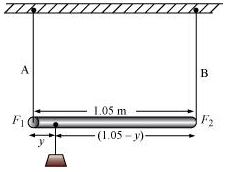## Pages

### Mechanical Properties of solids NCERT Solutions Class 11 Physics - Solved Exercise Question 9.18

Question 9.18:
A rod of length 1.05 m having negligible mass is supported at its ends by two wires of steel (wire A) and aluminium (wire B) of equal lengths as shown in Fig. 9.15. The cross-sectional areas of wires A and B are 1.0 mm2 and 2.0 mm2, respectively. At what point along the rod should a mass m be suspended in order to produce (a) equal stresses and (b) equal strains in both steel and aluminium wires.Answer :
Cross-sectional area of wire A, a1 = 1.0 mm2 = 1.0 × 10–6 m2
Cross-sectional area of wire B, a2 = 2.0 mm2 = 2.0 × 10–6 m2
Young’s modulus for steel, Y1 = 2 × 1011 Nm–2
Young’s modulus for aluminium, Y2 = 7.0 ×1010 Nm–2
(a) Let a small mass m be suspended to the rod at a distance y from the end where wire A is attached.
Stress in the wire = Force / Area  =  F / a
If the two wires have equal stresses, then:
F1 / a1  =  F2 / a2
Where,
F1 = Force exerted on the steel wire
F2 = Force exerted on the aluminum wire
F1 / F2 = a1 / a2  =  1 / 2    ....(i)
The situation is shown in the following figure:Taking torque about the point of suspension, we have:
F1y = F2 (1.05 - y)
F1 / F2 = (1.05 - y) / y    ......(ii)
Using equations (i) and (ii), we can write:
(1.05 - y) / y  = 1 / 2
2(1.05 - y)  =  y
y = 0.7 m
In order to produce an equal stress in the two wires, the mass should be suspended at a distance of 0.7 m from the end where wire A is attached.
(b) Young's modulus = Stress / Strain
Strain = Stress / Young's modulus  =  (F/a) / Y
If the strain in the two wires is equal, then:
(F1/a1) / Y1  =  (F2/a2) / Y2
F1 / F2 = a1Y1 / a2Y2
a1 / a2 = 1 / 2
F1 / F2 = (1 / 2) (2 X 1011 / 7 X 1010)  =  10 / 7      .......(iii)
Taking torque about the point where mass m, is suspended at a distance y1 from the side where wire A attached, we get:
F1y1 = F2 (1.05 – y1)
F1 / F2  =  (1.05 - y1) / y1    ....(iii)
Using equations (iii) and (iv), we get:
(1.05 - y1) / y1  =  10 / 7
7(1.05 - y1)  =  10y1
y1 = 0.432 m
In order to produce an equal strain in the two wires, the mass should be suspended at a distance of 0.432 m from the end where wire A is attached.